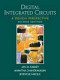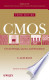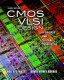Digital Microelectronic Circuits

Course Description

Introduction. CMOS digital circuits devices. MOS transistor models. CMOS process layout rules. CMOS inverter - static and dynamic characteristics, power. Combinational CMOS logic circuits - complementary, ratioed, pass-transistor, dynamic circuits. Sequential CMOS circuits - latches and flip-flops. Wires and interconnections - parameters and models, lines distribution problems. Arithmetical CMOS circuits - adders, multipliers, shifters. Memory CMOS circuits - layout organization, memory core circuit implementations, peripheral circuits. Input/output circuits.

General Competencies

Understanding of specific CMOS digital microelectonic circuits. Adoption of layout and electrical design methods for digital microelectronic cicuits.

Learning Outcomes

1. distinguish the role of individual layers of technological structure in the realization of CMOS circuits
2. identify different types of CMOS digital circuits
3. analyze basic CMOS digital circuits
4. arrange the properties of CMOS circuit with the transistor dimensions
5. evaluate the behavior of digital CMOS circuits
6. compare the properties of certain types of CMOS digital circuits
7. choose the kind of basic CMOS circuits to implement complex system
8. evaluate the justification for the integration of digital systems on a chip

Forms of Teaching

Lectures

lectures

Consultations

consultation

Seminars

seminars

Continuous Assessment Exam
Seminar/Project 0 % 30 % 0 % 30 %
Final Exam: Written 0 % 30 %
Final Exam: Oral 40 %
Exam: Written 0 % 30 %
Exam: Oral 40 %

Week by Week Schedule

1. Introduction. Microelectonic circuits evolution. CMOS manufacturing process.
2. CMOS devices. MOS transistor (current-voltage characteristics, parasitic).
3. MOS transistor scaling, MOS transistor SPICE models, MOS transistor model for manual analysis of digital circuits, Other CMOS process devices. CMOS inverter - basic properties. CMOS inverter static analysis (transfer characteristic, noise margins).
4. CMOS inverter dynamic analysis (parasitic capacitances, equivalent resistaneces, propagation delay minimization). CMOS inverter power dissipation.
5. Interconnect. Interconect wire parameters (capacitance, resistance, inductance). Electrical wire models (lumped C model, lumped RC model, distributed rc line, transmision line). Wire scaling.
6. Clock and power lines distribution problems. Combinational CMOS circuits. Complementary combinational CMOS circuits. NAND, NOR and complex logic function implementation.
7. Complementary CMOS circuits optimization. Ratioed logic circuits (pseudo-NMOS), Pass-Transistor CMOS circuits.
8. Midterm
9. Dynamic CMOS combinational circuits (Domino logic). Sequential CMOS circuits, Basic latch implementation, Multiplexer-based static sequential CMOS circuits.
10. Static flip-flops. Dynamic CMOS sequential circuits. Clock problems. Clock-skew insensitive circuits.
11. Aternative register styles. Nonbistable Seqential Circuits (Schmitt trigger, monostable and astable circuits). CMOS memory circuts. Memory classification. Memory layout organization.
12. RAM core circuit implementation (static and dynamic memory). Memory peripheral circuits.
15. CMOS multipliers (multiplication definition), CMOS multiplier implementations. Shifters.

Study Programmes

Electronics (profile)
Theoretical Course (2. semester)

Literature

Ž. Butković (2014.), Digitalni mikroelektronički sklopovi, Fakultet elektrotehnike i računarstva, Zagreb - interna skriptaJ.M. Rabaey, A. Chandrakasan, B. Nikolić (2003.), Digital Integrated Circuits - A Design Perspective, 2nd ed., Prentice HallR.J. Baker (2010.), CMOS - Circuit Design, Layout, and Simulation, 3rd ed., IEEE Press & Wiley-InterscienceN.H. E. Weste, D. Harris (2011.), CMOS VLSI Design - A Circuits and Systems Perspective, 4th ed., Addison-Wesley

General

ID 34549
Summer semester
5 ECTS
L1 English Level
L1 e-Learning
45 Lectures